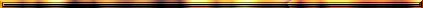.A|B|C|D|E|F|G|H|I|J|K|L|M|N|O|P|Q|R|S|T|U|V|W|X|Y|Z

O

Ocean of Causes: see K â r a n a ocean.

Om(kâra): or AUM, the p r a n â v a, the highest combination of syllables of which the letters represent the fundamental trinity of g u n a s, l o k a s, v e d a s, levels of realization, names, k l e s' a s etc.

- Soundvibration which represents the Absolute Truth, S' r î  K r i s h n a; it vibrates along in the H a r e  K r i s h n a - m a n t r a, to which it is subordinate.

- The holy sound OM, with which many vedic mantras begin, and which represents the Supreme Lord (see B.G. 9: 17, 8: 13 and 17: 24 and S.B 7.15: 31, 9.14: 48, 11.14: 34-35, 11.21: 36-40 and 12.6: 39-42).

- K r i s h n a's advice is to say three times a day ten times AUM for one's meditation so as to restore the p r â n a in a month's time (zie 11.14: 35).

Om tat sat: m a n t r a representing the Absolute Truth, God, the Supreme person. The G î t â explains: 17: 23.

Sanskrit Dictionary